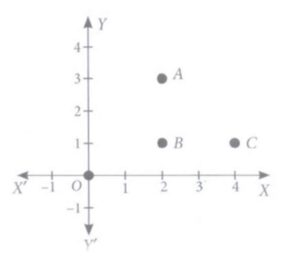Q) Alia and Shagun are friends living on the same street in Patel Nagar. Shagun’s house is at the
intersection of one street with another street on which there is a library. They both study in
the same school and that is not far from Shagun’s house. Suppose the school is situated at
the point O, i.e., the origin, Alia’s house is at A. Shagun’s house is at B and library is at C.Based on the above information, answer the following questions.

(i) How far is Alia’s house from Shagun’s house?
(ii) How far is the library from Shagun’s house?
(iii) Show that for Shagun, school is farther compared to Alia’s house and library.
OR
(iii)Show that Alia’s house, shagun’s house and library for an isosceles right triangle.

Ans:

(i) Distance of Alia’s house from Shagun’s house:

Coordinates of A (Alia’s house) = (2,3)

Coordinates of B (Shagun’s house) = (2,1)

Distance between A and B:

== 2 units

Therefore, Alia’s house is 2 units away from Shagun’s house.

(ii) Distance of library from Shagun’s house:

Coordinates of B (Shagun’s house) = (2,1)

Coordinates of C (Library) = (4,1)

Distance between B and C:

== 2 units

Therefore, Library is 2 units away from Shagun’s house.

(iii) A. Comparison of  Shagun’s distance from school, Alia’s house and library:

Coordinates of O (School) = (0,0)

Coordinates of B (Shagun’s house) = (2,1)

Distance between B and O:

=units

From answers (i) and (ii) above, we have

Distance of Shagun’s House from Alia’s house, BA = 2 units

and

Distance of Shagun’s House from Library, BC = 2 units

Since> 2 units,

therefore,  Shagun’s distance from school is farther as compared to Alia’s house and library:

(iii) B. Isosceles right angle triangle among Alia’s house, Shagun’s house and library:

Alia’s house, Shagun’s house and library form a triangle.

As we calculated in above answers that,

Distance of Shagun’s House from Alia’s house, BA = 2 units and

Distance of Shagun’s House from Library, BC = 2 units

Therefore, these three coordinates form an isosceles triangle,… Proved !

Next, to form right angle triangle, the should satisfy Pythagorean relationship:

Let’s calculate, distance of Alia’s House from Library:

Coordinates of A (Alia’s house) = (2,3)

Coordinates of C (Library) = (4,1)

Distance between A and C =units

Here, to satisfy Pythagorean relationship, we should if:

AB2 + BC2 = AC2

Let’s check for LHS:

AC = 2 units …………..calculated in part (i)

BC = 2 units …………. calculated in part (ii)

Therefore, LHS = (2)2 + (2)2 = 8 units

RHS: AC2 = (2√2)2 = 8 units

Since LHS = RHS,

therefore, triangle ABC is a right-angled triangle…… Proved !

Therefore, it is proved that Alia’s house, Shagun’s house and library form an Isosceles right angle triangle.

Scroll to Top